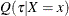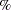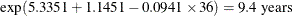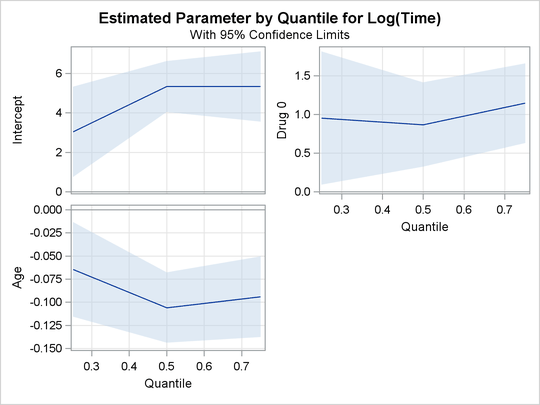# The QUANTLIFE Procedure (Experimental)

## Getting Started: QUANTLIFE Procedure

This example uses the human immunodeficiency virus (HIV) study data from Hosmer and Lemeshow (1999) to illustrate the basic features of PROC QUANTLIFE.

In this study, subjects were followed after a confirmed diagnosis of HIV. The primary goal was to evaluate the effect of various factors on the survival time. Two covariates for each subject were collected: age and history of prior intravenous drug use.

The following DATA step creates the data set HIV, which contains the variable Time (the follow-up time in days), the variable Status (with value 0 if Time was censored and 1 otherwise), the variable Drug (with value 1 for prior intravenous drug use and 0 otherwise), and the variable Age (the patient’s age in years at the beginning of the follow-up).

data HIV;
input Time Age Drug Status;
datalines;
5      46      0        1
6      35      1        0
8      30      1        1
3      30      1        1
22      36      0        1
1      32      1        0

... more lines ...

1      34      1        1
;


You can use PROC QUANTLIFE to explore the relationship between the survival time and the two covariates at different quantiles.

Suppose you are interested in the median survivors and in the longer and shorter survivors. The following statements fit a linear model for the 25th, 50th, and 75th percentiles:

ods graphics on;
proc quantlife data=hiv log plots=quantplot seed=1268;
class Drug;
model Time*Status(0) = Drug Age / quantile=(0.25 0.5 0.75);
Drug_Effect: test Drug;
run;



The LOG option fits a quantile regression model for the log of Time, as is done by an accelerated failure time (AFT) model in standard survival analysis. The SEED= option is specified to maintain reproducibility of the resampling method that is used for statistical inference.

The MODEL statement specifies the response variable, Time, and the censoring variable, Censor. The value that indicates censoring is enclosed in parentheses. The values of Time are considered to be censored if the value of Censor is 0; otherwise, they are considered to be event times. The QUANTILE= option requests a fit of the conditional quantile functionat the quantile levels 0.25, 0.5, and 0.75.

The TEST statement requests a test for the hypothesis that there is no Drug effect at each of the quantile levels.

Figure 76.1 displays basic model information. For example, you can see from Figure 76.1 that the response is log(Time), and the censoring rate is 20%.

Figure 76.1: Model Fitting Information

The QUANTLIFE Procedure

Model Information
Data Set WORK.HIV
Dependent Variable Log(Time)
Censoring Variable Status
Censoring Value(s) 0
Number of Observations 100
Method Kaplan-Meier
Replications 200
Seed for Random Number Generator 1268

Class Level Information
Name Levels Values
Drug 2 0 1

Summary of the Number of Event and Censored
Values
Total Event Censored Percent
Censored
100 80 20 20.00

Figure 76.2 displays the parameter estimates, which are computed using the default Kaplan-Meier-type estimator. See the section Kaplan-Meier-Type Estimator for Censored Quantile Regression for details. In addition, Figure 76.2 displays standard errors, 95confidence limits, t values, and p-values that are computed by the default resampling method, exponentially weighted resampling. See the section Exponentially Weighted Method.

A different quantile regression model is fitted for each quantile, and the first column (Quantile) in Figure 76.2 identifies the model for the parameter estimates. Age has a negative effect on survival time. You can use the parameter estimates to predict the survival time at the quantiles of interests. For example, the 75th percentile survival time for a person with no previous IV drug use at age 45 isFigure 76.2: Parameter Estimates

Parameter Estimates
Quantile Parameter   DF Estimate Standard
Error
95% Confidence Limits t Value Pr > |t|
0.2500 Intercept   1 3.0373 1.1680 0.7482 5.3265 2.60 0.0108
Drug 0 1 0.9516 0.4403 0.0887 1.8146 2.16 0.0331
Drug 1 0 0 0 0 0 . .
Age   1 -0.0646 0.0261 -0.1158 -0.0135 -2.48 0.0150
0.5000 Intercept   1 5.3351 0.6605 4.0406 6.6296 8.08 <.0001
Drug 0 1 0.8681 0.2786 0.3219 1.4142 3.12 0.0024
Drug 1 0 0 0 0 0 . .
Age   1 -0.1059 0.0194 -0.1439 -0.0679 -5.46 <.0001
0.7500 Intercept   1 5.3351 0.9091 3.5532 7.1170 5.87 <.0001
Drug 0 1 1.1451 0.2625 0.6307 1.6596 4.36 <.0001
Drug 1 0 0 0 0 0 . .
Age   1 -0.0941 0.0223 -0.1378 -0.0505 -4.23 <.0001

The PLOTS=QUANTPLOT option in the PROC QUANTLIFE statement requests the quantile process plots, which are shown in Figure 76.3. The quantile process plot is a scatter plot of an estimated regression. Figure 76.3 shows that the estimated coefficients for the two covariates does not change much across quantiles.

Figure 76.3: Estimated ParametersThe tests that are requested by the TEST statement are shown in Figure 76.4.

Figure 76.4: Tests of Significance

Test Drug_Effect Results
Quantile DF Chi-Square Pr > ChiSq
0.2500 1 4.67 0.0307
0.5000 1 9.70 0.0018
0.7500 1 19.03 <.0001

The tests indicate that the coefficient of Drug is significantly different from 0 at the 25th, 50th, and 75th percentiles.